### Home > CALC > Chapter 7 > Lesson 7.4.4 > Problem7-207

7-207.
1.No calculator! Examine the integrals below. Consider the multiple tools available for integrating and use the best strategy. After evaluating each integral, write a short description of your method. Homework Help ✎

1.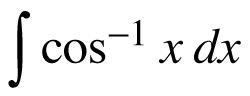2.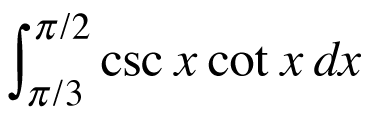3.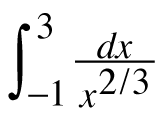4.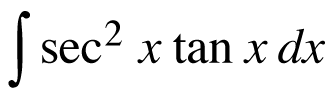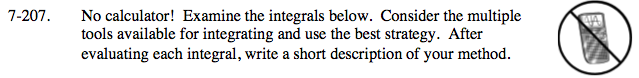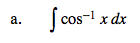$f=\text{cos}^{-1}x, \ dg=dx, \ df=-\frac{1}{\sqrt{1-x^{2}}}dx, \ g=x$

$\int \text{cos}^{-1}xdx=x\text{cos}^{-1}x+\int \frac{xdx}{\sqrt{1-x^{2}}}$

Use integration by parts and substitution.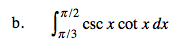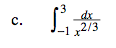There is a vertical asymptote at x = 0, so this improper integral needs to be rewritten in proper form (as a limit).

In fact, write a sum of two proper integrals.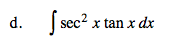Use u-substitution. sec2xtanx = (secx)(secx)(tanx)